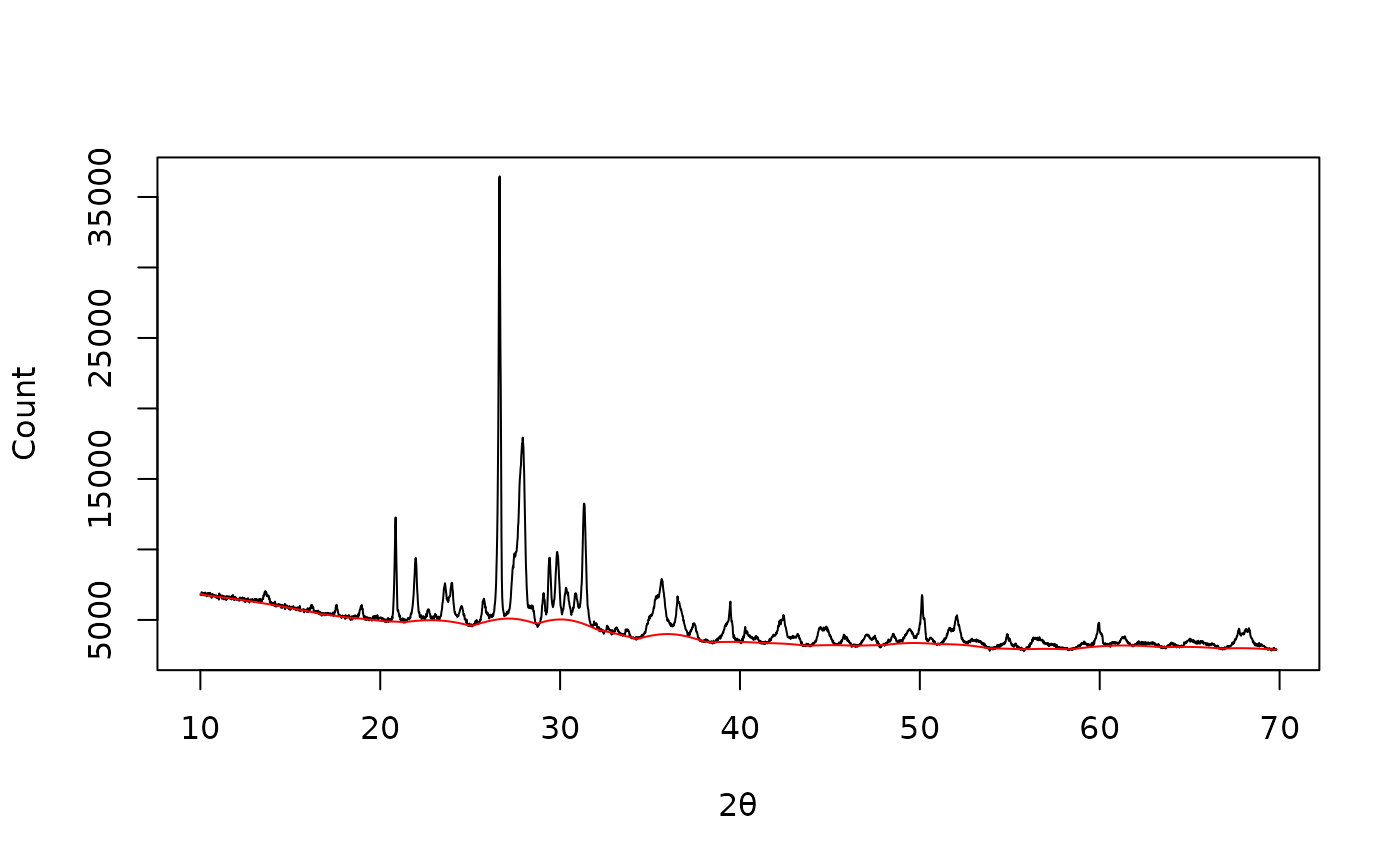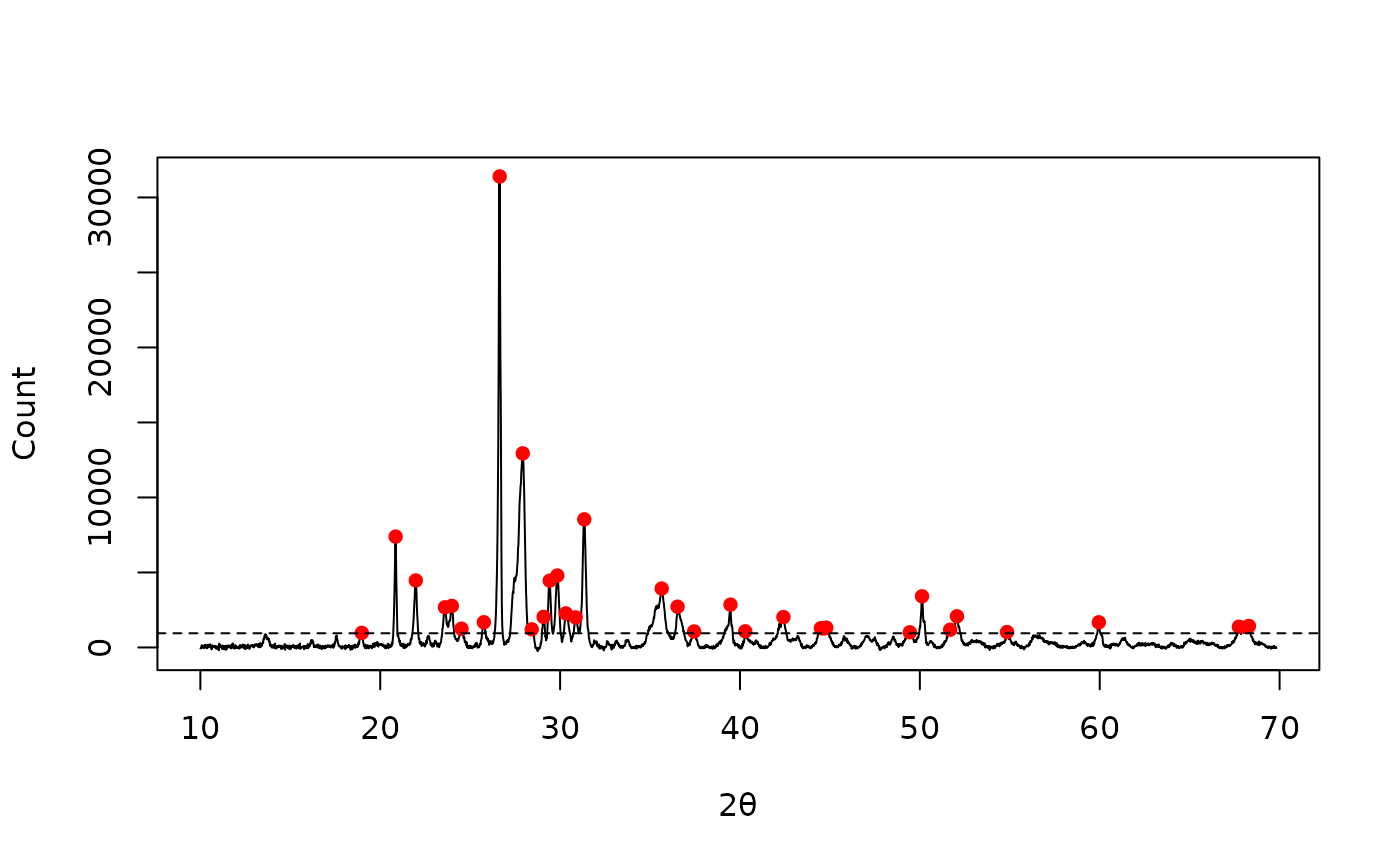Finds local maxima in sequential data.

## Usage

peaks_find(x, y, ...)

# S4 method for numeric,numeric
peaks_find(x, y, method = "MAD", SNR = 2, m = NULL, ...)

# S4 method for ANY,missing
peaks_find(x, method = "MAD", SNR = 2, m = NULL, ...)

## Arguments

x, y

A numeric vector. If y is missing, an attempt is made to interpret x in a suitable way (see grDevices::xy.coords()).

...

Extra parameters to be passed to internal methods.

method

A character string specifying the method to be used for background noise estimation (see below).

SNR

An integer giving the signal-to-noise-ratio for peak detection (see below).

m

An odd integer giving the window size (i.e. the number of adjacent points to be used). If NULL, 5% of the data points is used as the half window size.

## Value

Returns a list with two components x and y.

## Details

A local maximum has to be the highest one in the given window and has to be higher than $$SNR \times noise$$ to be recognized as peak.

The following methods are available for noise estimation:

MAD

Median Absolute Deviation.

Note that to improve peak detection, it may be helpful to smooth the data and remove the baseline beforehand.

## Note

There will be $$(m - 1) / 2$$ points both at the beginning and at the end of the data series for which a complete $$m$$-width window cannot be obtained. To prevent data loss, progressively wider/narrower windows are used at both ends of the data series.

Adapted from Stasia Grinberg's findPeaks function.

Other peaks detection methods: peaks_fwhm()

N. Frerebeau

## Examples

## X-ray diffraction
data("XRD")

## Whittaker smoothing
smooth <- smooth_whittaker(XRD, lambda = 1000, d = 2, sparse = TRUE)

## 4S Peak Filling baseline
baseline <- baseline_peakfilling(smooth, n = 10, m = 5, by = 10)

plot(XRD, type = "l", xlab = expression(2*theta), ylab = "Count")
lines(baseline, type = "l", col = "red")## Correct baseline
XRD <- signal_drift(XRD, lag = baseline, subtract = TRUE)

## Find peaks
peaks <- peaks_find(XRD, SNR = 3, m = 11)

plot(XRD, type = "l", xlab = expression(2*theta), ylab = "Count")
lines(peaks, type = "p", pch = 16, col = "red")
abline(h = attr(peaks, "noise"), lty = 2) # noise threshold## Half-Width at Half-Maximum
x <- seq(-4, 4, length = 1000)
y <- dnorm(x)

peaks_fwhm(x, y, center = 0) # Expected: 2 * sqrt(2 * log(2))
#>  2.354354